# “Never Calculate without Already Knowing the Answer!” by Yuliya K. '18

xTalk about the "Art of Insight" by Prof Sanjoy Mahajan

If you've been to a high school math class recently, you probably remember seeing an inordinate number of calculators. You might’ve also observed students entering anything from 999*999 to sin(π/6) to 7-1 into the machines. The calculator habit is so strong that even 5+7 (=12) warrants machine help. And habits die hard.

To combat our calculator obsession, Sanjoy Mahajan suggests a radical departure from the calculator addiction: What if we knew the solution before the calculation? In Prof Mahajan’s words, “Never calculate without already knowing the answer!” Sounds bizarre, but let him explain, and you will be intrigued.

First, a brief introduction. Sanjoy Mahajan is an Associate Professor of Applied Science and Engineering at Olin College. At MIT, Prof Mahajan is also a familiar and welcome figure. He has collaborated with MIT professors to improve their curricula and published a book, Street-Fighting Mathematics, at the MIT Press (read the book here).

Sanjoy Mahajan sees a general trend of pragmatism in the United States, with students wishing to just solve the problem without asking the essential “how” or “why.” Science problems then become nothing more than a repeated and calculator-assisted applications of a single formula. Unfortunately, even the most complex formulas can be wrong. Calculators can’t reveal that, but a better understanding, or insight, of the material can. In his May 19 xTalk “The Art of Insight,” hosted by the MIT Office of Digital Learning, Prof Mahajan used several examples to illustrate the benefits of getting to the solution before reaching for a calculator.

EXAMPLE #1: consider being in a room with a paper towel freshly sprayed with perfume (as during Prof Mahajan’s talk). The question: how long does it take for all audience members to smell the aroma?

Formal Model:
Formally, the answer to that,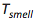, is derived with a diffusion equation which, according to Prof Mahajan, is a “big mess” that requires knowledge of Calculus. Furthermore, the solved equation tells us thatis 10^7 seconds, or approximately ⅓ a year, while, in real life, the back rows smelled the perfume after 30 or so seconds! The official, calculator-enabled, model for the problem is way off. Despite the complexity, it fails to provide a reasonable answer.

Insight:
Add to the consideration “a four-letter word”: wind. With wind, or drift, you can start taking much bigger, though slower, steps to a solution instead of the small and fast steps of the diffusion model. This common-sense model has the benefit of search control: making search space much more tractable by expanding it.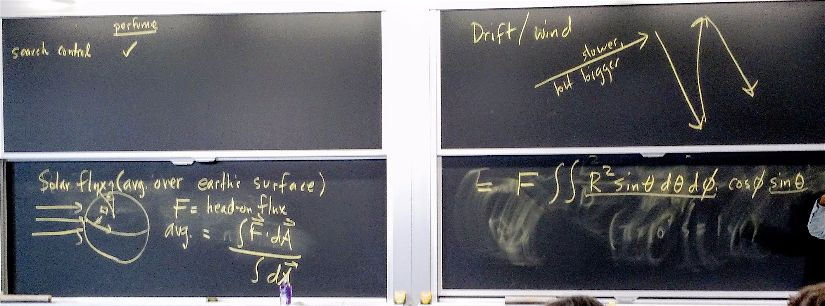EXAMPLE #2: calculate the average solar flux,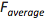, over the Earth’s surface.

Formal Model:
Again, the formal solution requires Calculus, more specifically integration. It’s complicated (so much so, in fact, that I fear to reproduce it in this post).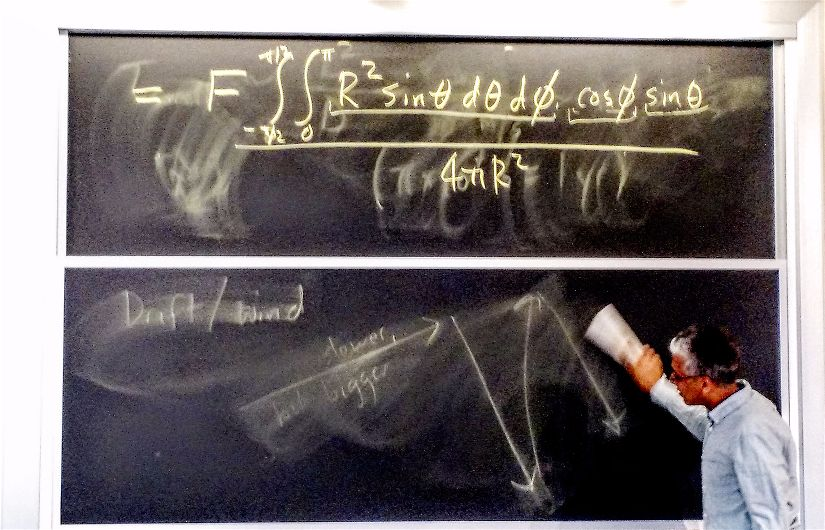Insight:
The “knowing the answer,” or the intuitive, method considers only the solar flux over a slice of the Earth to arrive at the same answer with a one-step calculation: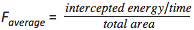.

The latter formula is easier to understand and much more robust. Plus it allows for simple modifications to account for the ellipsoid/tilted properties of the Earth (modifications that would look terrifying in the formal model). And if you understand the simpleformula, you can transfer the insight over to other topics, like the kinetic theory of gases.

Why is the visual method preferable here (remember, we looked at what happens on one “slice” of the Earth)? Turns out, we’ve been perceiving, or seeing, the world about 10^3 times longer than we’ve been able to talk about it! The visual cortex developed long before language, which is why it’s easier for us to think in visuals. Even Prof Mahajan’s most important word during the talk, “insight,” has the word “sight” built in. In fact, many languages contain “sight” within words that signify “understanding.” The lesson here: use your large visual cortex whenever you can.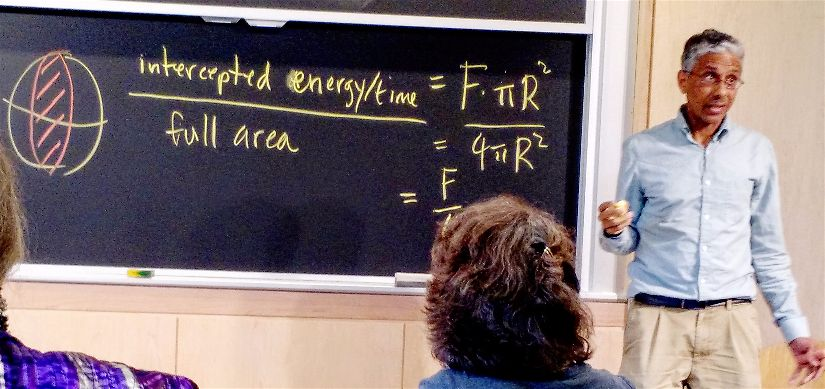EXAMPLE #3: Imagine you’re running away from “bad guys” and throw a stone in a nearby well to find out how deep it is. It takes T = 4 seconds for the stone to drop, with the speed of sound being the usual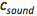= 340 m/s and gravitational constant, g, being 10 m/s^2. Question: roughly how deep is the well? Answer options: a) 20 m, b) 100 m, c) 500 m.

Formal Model:
Let’s consider the proper mathematical way of solving the problem first. To do this, we must include the time it takes for the sound to travel up the well (a factor we would normally not consider since it is so negligible in our scenario). We get a quadratic equation that derives at the following formula for the height of the well: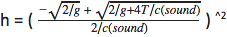.

A bit overwhelming, isn’t it? And certainly unhelpful in a high speed chase situation. According to Prof Mahajan, the answer is problematic for one specific reason: it has a high entropy.

In chemistry, the entropy for chemical substances is defined as entropy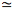log(# states), a sort of quantifier of how “messy” or “chaotic” the substance is. While we don’t normally think of entropy as applying to mathematical expressions, Prof Mahajan suggests that we can, in fact, measure the entropy of expressions by re-defining the formula from chemistry as entropylog(# of plausible versions of the expression).

Consider now how many ways we can rearrange the formula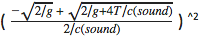by moving constants around the fraction bars and square roots (for example, one simple change would be to move theto the top of the large fraction bar). This mathematical expression has a high entropy of structure.

The problem with high-entropy expressions is that they carry a high cognitive load, which is how much working memory you need to use to process them. As humans, we have approximately infinite long-term memory. However, to store information in the long term, we need to get it through the limited working/short-term memory. There, we have serious cognitive limitations, and high-entropy expressions like the one above significantly increase the working memory load. Thus, entropy also measures how much information you can process at a time.

In short, according to Prof Mahajan, the rule of thumb is: if you get a quadratic expression in a problem, you did something wrong. The high entropy of quadratics is bad.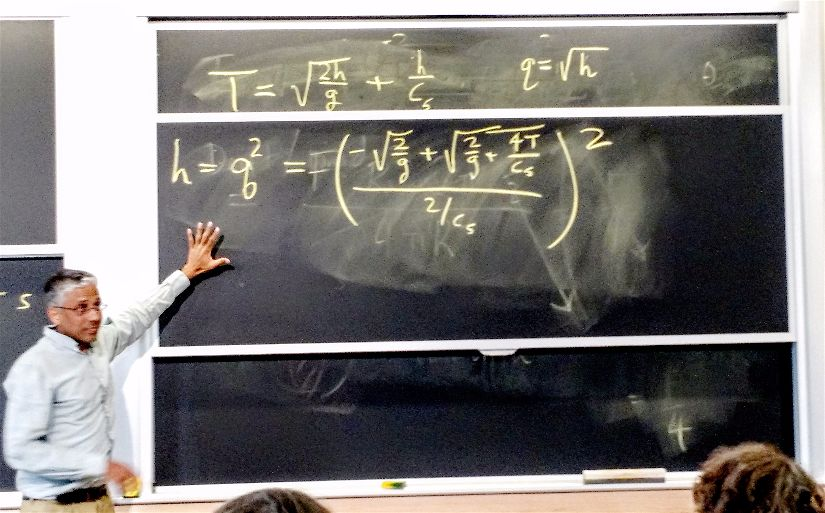Insight:

So what’s the easy way? By applying some real-world insight, we can neglect the “journey” of sound. Then h= ½ gT^2 ~ 80 m. Once you have the insight into the problem and a preliminary estimation, you can improve upon the result by considering t(sound) separately. Benefit of the insight here is low entropy/low cognitive load. Plus, there is no need for a calculator, a tool not readily available during a daring escape.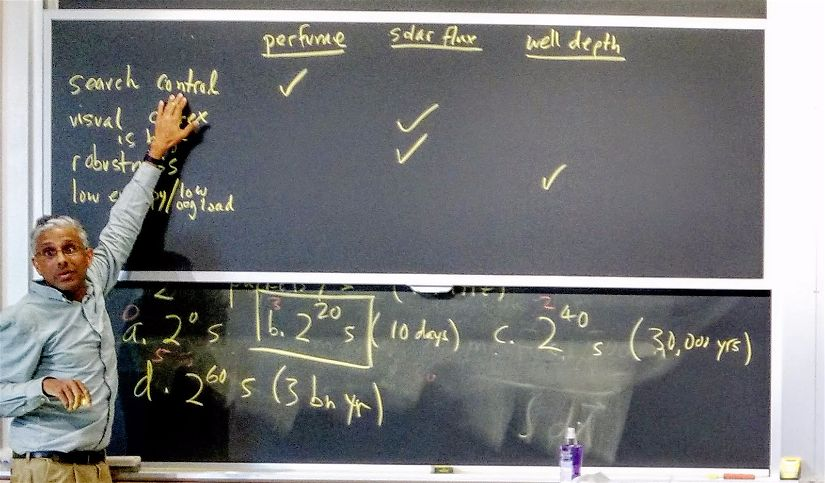You might say at this point that we have simply looked at three disjoint examples rather than the general idea: how we benefit from the Art of Insight. Let’s recap now to consider all the benefits of reasoning, rather than calculating, the solution.

1. Search control (making search space much more tractable by expanding it). Main example: perfume diffusion.
2. Transfer (to other problems). Main example: perfume diffusion.
3. Use of the (larger) visual cortex. Main example: solar flux.
4. Robustness. Main example: solar flux.
5. Low entropy/low cognitive load. Main example: well height.

Although I noted the “main” examples here, the five properties can be applied to all three problems. This is our “homework,” according to Prof Mahajan.